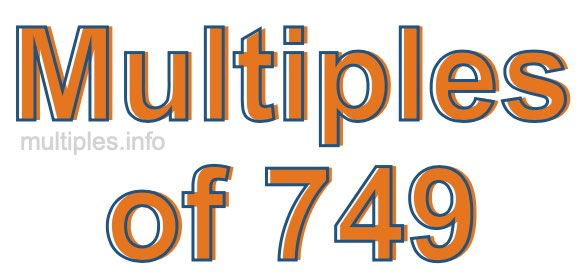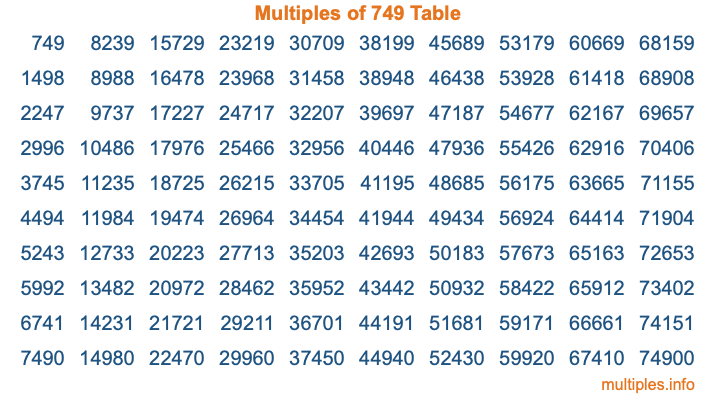Multiples of 749Welcome to the Multiples of 749 page. Here we will first teach you everything you will ever need to know about the multiples of 749, and then give you a study guide summary of everything we taught you to make sure you remember it all. Use this page to look up facts and learn information about the multiples of 749. This page will make you a multiples of seven hundred forty-nine expert!

Definition of Multiples of 749
Multiples of 749 are all the numbers that when divided by 749 equal an integer. Each of the multiples of 749 are called a multiple. A multiple of 749 is created by multiplying 749 by an integer.

Therefore, to create a list of multiples of 749, you start with 1 multiplied by 749, then 2 multiplied by 749, then 3 multiplied by 749, and so on for as long as you want. Thus, the list of the first five multiples of 749 is 749, 1498, 2247, 2996, and 3745. To see a larger list of multiples of 749, see the printable image of Multiples of 749 further down on this page. We also have a category where you can choose any nth multiple of 749.

Multiples of 749 Checker
The Multiples of 749 Checker below checks to see if any number of your choice is a multiple of 749. In other words, it checks to see if there is any number (integer) that when multiplied by 749 will equal your number. To do that, we divide your number by 749. If the the quotient is an integer, then your number is a multiple of 749.

Is  a multiple of 749?

Least Common Multiple of 749 and ...
A Least Common Multiple (LCM) is the lowest multiple that two or more numbers have in common. This is also called the smallest common multiple or lowest common multiple and is useful to know when you are adding our subtracting fractions. Enter one or more numbers below (749 is already entered) to find the LCM.

Check out our LCM Calculator if you need more details about the Least Common Multiple or if you need the LCM for different numbers for adding and subtraction fractions.

nth Multiple of 749
As we stated above, 749 is the first multiple of 749, 1498 is the second multiple of 749, 2247 is the third multiple of 749, and so on. Enter a number below to find the nth multiple of 749.

th multiple of 749

Multiples of 749 vs Factors of 749
749 is a multiple of 749 and a factor of 749, but that is where the similarities end. All postive multiples of 749 are 749 or greater than 749. All positive factors of 749 are 749 or less than 749.

Below is the beginning list of multiples of 749 and the factors of 749 so you can compare:

Multiples of 749: 749, 1498, 2247, 2996, 3745, etc.

Factors of 749: 1, 7, 107, 749

As you can see, the multiples of 749 are all the numbers that you can divide by 749 to get a whole number. The factors of 749, on the other hand, are all the whole numbers that you can multiply by another whole number to get 749.

It's also interesting to note that if a number (x) is a factor of 749, then 749 will also be a multiple of that number (x).

Multiples of 749 vs Divisors of 749
The divisors of 749 are all the integers that 749 can be divided by evenly. Below is a list of the divisors of 749.

Divisors of 749: 1, 7, 107, 749

The interesting thing to note here is that if you take any multiple of 749 and divide it by a divisor of 749, you will see that the quotient is an integer.

Multiples of 749 Table
Below is an image of the first 100 multiples of 749 in a table. The table is in chronological order, column by column. The first column has the first ten multiples of 749, the second column has the next ten multiples of 749, and so on.The Multiples of 749 Table is also referred to as the 749 Times Table or Times Table of 749. You are welcome to print out our table for your studies.

Negative Multiples of 749
Although not often discussed or needed in math, it is worth mentioning that you can make a list of negative multiples of 749 by multiplying 749 by -1, then by -2, then by -3, and so on, to get the following list of negative multiples of 749:

-749, -1498, -2247, -2996, -3745, etc.

Multiples of 749 Summary
Below is a summary of important Multiples of 749 facts that we have discussed on this page. To retain the knowledge on this page, we recommend that you read through the summary and explain to yourself or a study partner why they hold true.

There are an infinite number of multiples of 749.

A multiple of 749 divided by 749 will equal a whole number.

749 divided by a factor of 749 equals a divisor of 749.

The nth multiple of 749 is n times 749.

The largest factor of 749 is equal to the first positive multiple of 749.

749 is a multiple of every factor of 749.

749 is a multiple of 749.

A multiple of 749 divided by a divisor of 749 equals an integer.

749 divided by a divisor of 749 equals a factor of 749.

Any integer times 749 will equal a multiple of 749.

Multiples of a Number
Here you can get the multiples of another number, all with the same attention to detail as we did for multiples of 749 on this page.

Multiples of
Multiples of 750
Did you find our page about multiples of seven hundred forty-nine educational? Do you want more knowledge? Check out the multiples of the next number on our list!

Copyright  |   Privacy Policy  |   Disclaimer  |   Contact